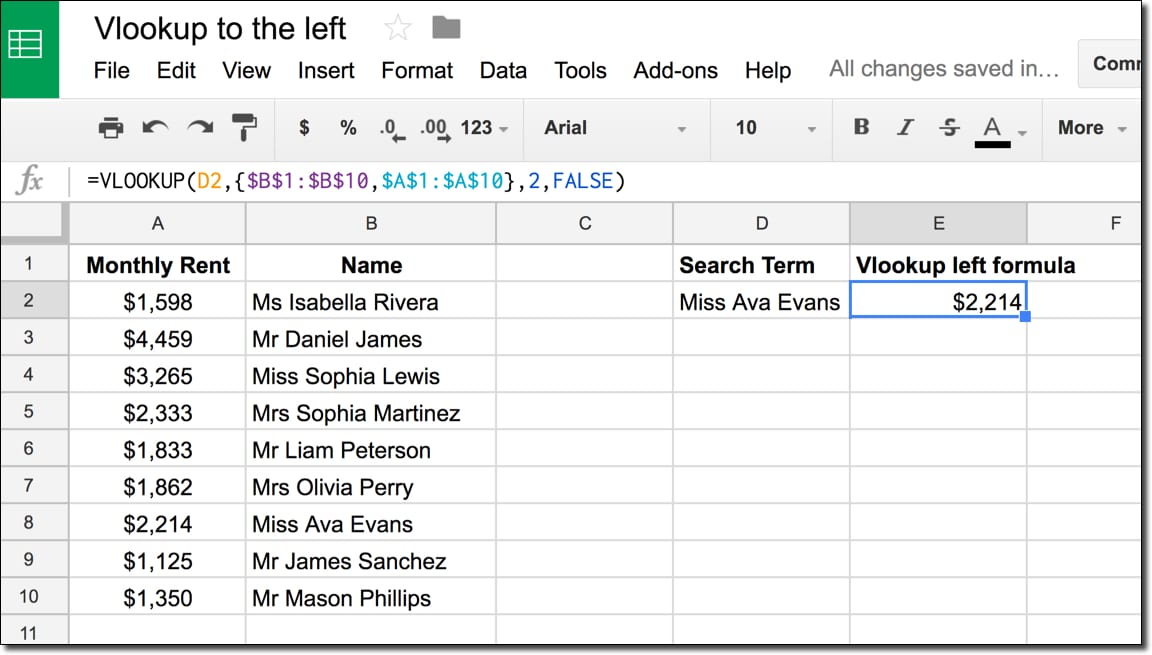## Reverse Text In Google Sheets

In this example, you’ll see how to reverse text in Google Sheets.

To start, enter some text into cell A1 and the formula in cell B1, to reverse the order of the text: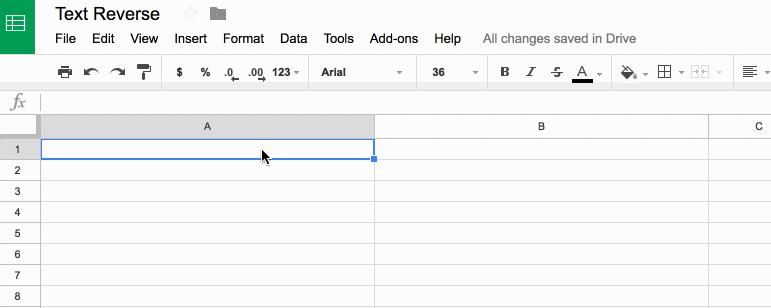## Reverse Text In Google Sheets

### What’s the formula?

`=ArrayFormula(IFERROR(PROPER(CONCATENATE(MID(A1,LEN(A1)-ROW(INDIRECT("1:"&LEN(A1)))+1,1))),""))`

It’s a beast! See below for a detailed breakdown of how it works.

It might be useful if you wanted to find the last character in a string, or the last occurrence of a character using the FIND() function.

Here’s an alternative, which reverses the capitalization too (submitted by Michael D over email – thanks!):

`=JOIN("",ARRAYFORMULA(MID(A1,LEN(A1)-ROW(INDIRECT("1:"&LEN(A1)))+1,1)))`

### How does this formula work?

Basically we make an array of numbers corresponding to how many letters are in the original text string. Then we reverse that, so the array shows the largest number first. Then we extract each letter at that position (so the largest number will extract the last letter, the second largest will extract the second-to-last letter, etc., all the way to the smallest number extracting the first letter). Then we concatenate these individual letters.

Easy! Err…

The only way to really understand this formula is to break it down, starting from the inner functions and building back out.

Assuming we have the text string “Abc” in cell A1, then let’s build the formula up in cell B1, step-by-step:

Step 1:

Use the LEN function to calculate the length of the text string and turn it into a range string with “1:”&LEN(A1)

Use the INDIRECT function to turn this string range reference into a valid range reference.

Finally wrap with ROW to convert into a row number list.

`=ROW(INDIRECT("1:"&LEN(A1)))`

which outputs a result of 1 in cell B1.

Step 2:

Turn the formula into an array formula, by hitting Ctrl + Shift + Enter, or Cmd + Shift + Enter (on a Mac), to the formula above. This will add the `ArrayFormula` wrapper around the formula:

`=ArrayFormula(ROW(INDIRECT("1:"&LEN(A1))))`

This outputs 1 in cell B1, 2 in cell B2 and 3 in cell B3: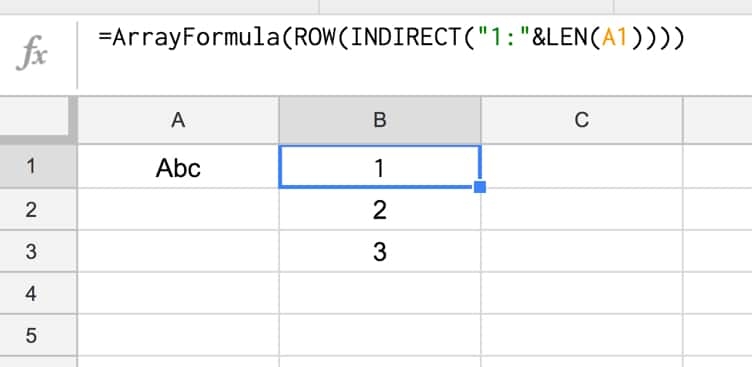Step 3:

Reverse the output, so 3 is in cell B1, 2 in B2 and 1 in B3, by subtracting from the length of the text in A1 and adding 1 to avoid 0:

`=ArrayFormula(LEN(A1)-ROW(INDIRECT("1:"&LEN(A1))) + 1)`

Step 4:

Use the MID formula to now extract the letters at position 3 (“c”), position 2 (“b”) and position 1 (“A”) and display in cells B1:B3:

`=ArrayFormula(MID(A1,LEN(A1)-ROW(INDIRECT("1:"&LEN(A1))) + 1 , 1))`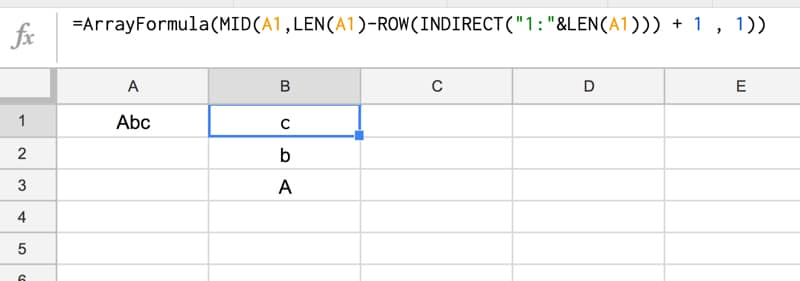Step 5:

Concatenate so that all the individual outputs are combined into a single cell:

`=ArrayFormula(CONCATENATE(MID(A1,LEN(A1)-ROW(INDIRECT("1:"&LEN(A1))) + 1 , 1)))`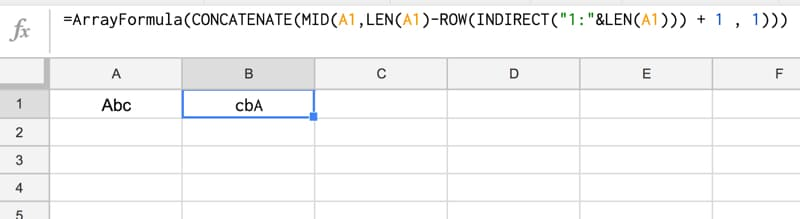Step 6 (optional):

The string is essentially reversed now, so we could stop here.

However, you can use the PROPER function to capitalize the first letters of each word only, for a true reverse effect:

`=ArrayFormula(PROPER(CONCATENATE(MID(A1,LEN(A1)-ROW(INDIRECT("1:"&LEN(A1))) + 1 , 1))))`

Step 7 (optional):

Last step is to add in an IFERROR function to avoid an unsightly error message if the input cell (A1) is blank:

`=ArrayFormula(IFERROR(PROPER(CONCATENATE(MID(A1,LEN(A1)-ROW(INDIRECT("1:"&LEN(A1)))+1,1))),""))`

Final output:

Here’s the formula in action again: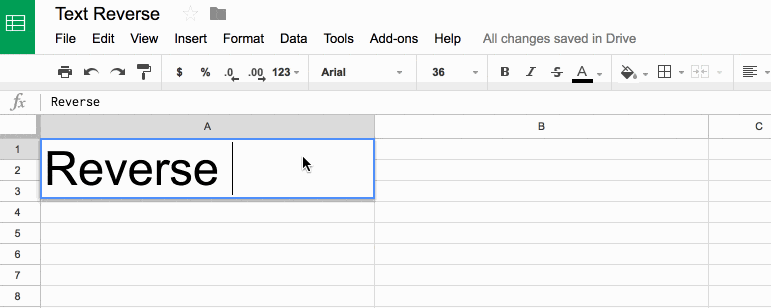## How To Combine Text And Numbers In Google Sheets

This tutorial will show you how to combine text and numbers in Google Sheets, and keep the the correct number formatting in place.

Combining text and numbers is common in spreadsheets, for example when you want to add some context, e.g.:

Total: \$5,250
Discount: 35.5%

However, if you try to combine text with numbers (or currencies/percentages/dates) directly in a cell in Google Sheets, you’ll notice the numbers loses any formatting, which will make the number harder to read or even less meaningful, e.g.:

Total: 5250
Discount: 0.355

You can use the TEXT function to fix this, and apply formatting to numbers that are displayed with text in a cell.

## First And Last Days Of The Prior Month In Google Sheets?

In this example, we’re going to see how to extract the first and last dates of the prior month, i.e. the last full month before this current one.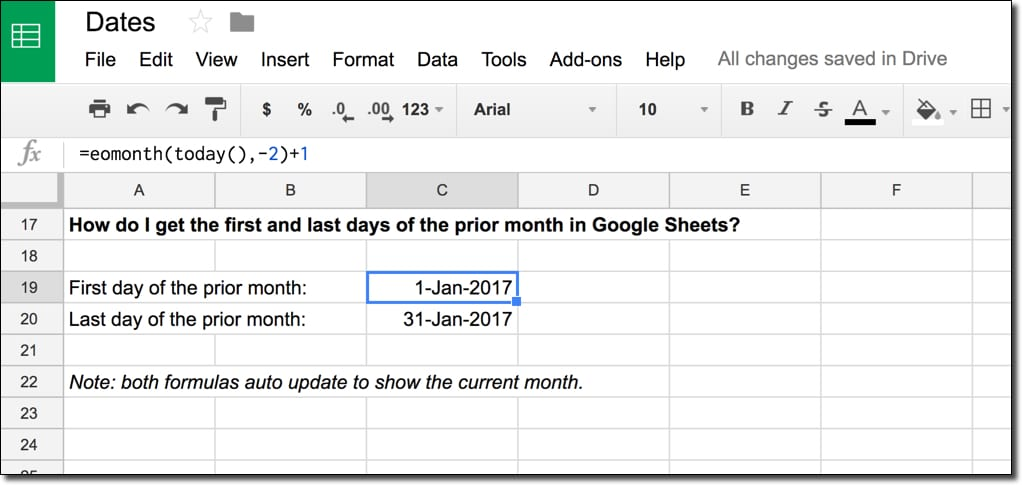It’s the sort of date filter that’s frequently used in digital marketing, when you want to restrict your data to just web traffic or revenue in this period for comparison.

## How do I get the first and last dates of the previous month?

We combine two functions, TODAY and EOMONTH, and a little bit of math to create the formulas to extract first and last dates.

### What’s the formula?

For the first day of the prior month:

`=EOMONTH(TODAY(),-2)+1`

For the last day of the prior month:

`=EOMONTH(TODAY(),-1)`

### How does this formula work?

The heart of both of these formulas is the function TODAY(), which outputs today’s date in your Sheet. It updates automatically whenever the spreadsheet is recalculated (when you make an edit somewhere else). It’s known as a volatile function because it automatically recalculates, so if you were to have a huge number of these formulas, it would affect the performance of your spreadsheet.

`=TODAY()`

Next we wrap that with the EOMONTH function to get the date at the end of the month.

For the first day of the previous month, we offset by -2 to go back two months (which gives the last day of December in this example). To this we then add 1 day to nudge us into the first day of the previous month (January 2017 in this example), as follows:

`=EOMONTH(TODAY(),-2)+1`

The formula for the last day of the prior month is simpler. We offset -1 to go back one month and we don’t need to add a day to the output. So the formula is:

`=EOMONTH(TODAY(),-1)`

These types of date formulas are super useful if you do any data analysis work, and want to group and compare data for set periods. As an example, you may want to automatically generate Start and End Date fields in the Google Analytics Add-On, to always extract the most recent data for current and prior month periods for comparison.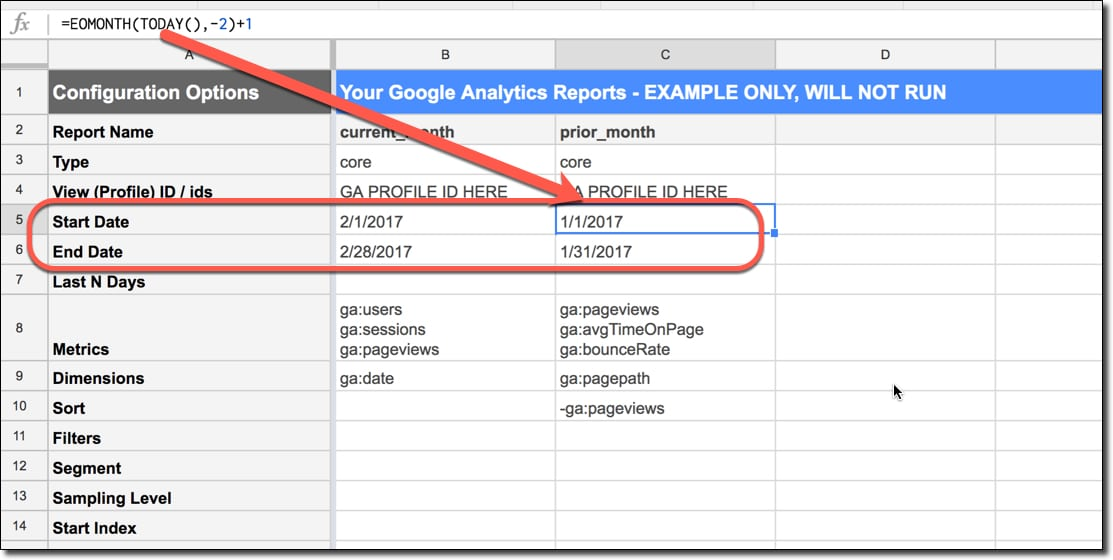See also: We can perform similar calculations to get weekly dates, prior months, quarterly dates and yearly dates.

## Have Vlookup Return Multiple Columns in Google Sheets

Have you ever wished the VLOOKUP function could return multiple columns?

For example, maybe you want to return several values that match a search term so that you can use a range of data as an input for another formula (e.g. a sparkline).Of course, you could set up multiple vlookup formulas, but this wouldn’t work if you want to pass the data range into another formula, like the sparkline for example.

However, you can easily achieve it with just one formula.

## How To VLOOKUP To The Left In Google Sheets?

The VLOOKUP function is a super popular formula but suffers from a major drawback. You can’t lookup data to the left!

However, there’s a sneaky trick that lets us VLOOKUP to the left, so we can search for a term and return a result from a column to the left of the original search column: